## 2.13Incompressible ﬂow

We can derive a set of equations for incompressible ﬂow that includes:

• mass conservation, Eq. (2.8 );
• momentum conservation, Eq. (2.19 );
• the material derivative for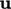, Eq. (2.26 );
• the rate of deformation tensor, Eq. (2.33 );
• the Newtonian ﬂuid model, Eq. (2.41 ).

Combining Eq. (2.41 ) and Eq. (2.33 ) and using Eq. (2.36 ) for the deviatoric part of a tensor, gives an expression for stress: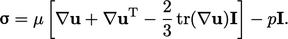(2.44)
Substituting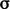into Eq. (2.19 ) and applying Eq. (2.26 ) gives an equation for momentum for a Newtonian ﬂuid: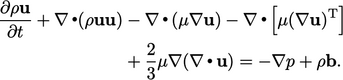(2.45)
The derivation of the equation above uses Eq. (2.35 ) and the identity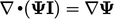on page 84 .

An incompressible ﬂuid exhibits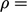constant over time, i.e. for moving volumes of ﬂuid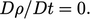Combining the material derivative Eq. (2.14 ) and mass conservation Eq. (2.8 ) gives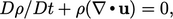which results in the incompressibility condition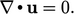(2.46)

A homogeneous, incompressible material exhibits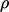= constant uniformly throughout the entire ﬂuid. With that assumption, Eq. (2.45 ) can be written as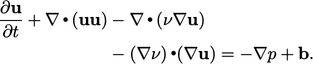(2.47)
This is the momentum equation for a homogeneous, incompressible Newtonian ﬂuid. It includes: kinematic viscosity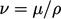in SI units of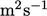; and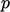represents kinematic pressure, i.e. divided by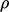, in SI units of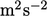.

The identity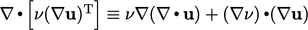and the incompressibility Eq. (2.46 ) yield the terms in Eq. (2.47 ).

### Pressure equation

Mass and momentum conservation, represented by Eq. (2.46 ) and Eq. (2.47 ) respectively, provide two equations — one scalar, one vector — for two ﬁelds,and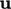. However, Eq. (2.46 ) cannot be solved in its own right since it provides only one equation for vector, containing 3 components.

A scalar equation, including bothand, can be derived by taking the divergence of Eq. (2.47 ) and eliminating terms by substituting Eq. (2.46 ), noting that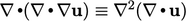. For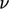and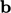that are constant and uniform, the equation is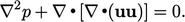(2.48)
This pressure equation can replace Eq. (2.46) to provide a pair of equations, with Eq. (2.47 ), for both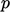and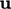. Chapter 5 describes the algorithms used in the ﬁnite volume method which couple the two equations using a modiﬁed form of Eq. (2.48 ).
Notes on CFD: General Principles - 2.13 Incompressible ﬂow